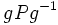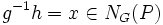# Frattini's argument

This article describes a fact or result that is not basic but it still well-established and standard. The fact may involve terms that are themselves non-basic
View other semi-basic facts in group theory
VIEW FACTS USING THIS: directly | directly or indirectly, upto two steps | directly or indirectly, upto three steps|
This article gives a proof/explanation of the equivalence of multiple definitions for the term automorph-conjugate subgroup
View a complete list of pages giving proofs of equivalence of definitions

## Statement

### For automorph-conjugate subgroups

Let$H$ be a normal subgroup of$G$ and$P$ an automorph-conjugate subgroup of$H$. Then:$HN_G(P) = G$

where$N_G(P)$ denotes the normalizer of$P$ in$G$.

### For Sylow subgroups

Let$H$ be a normal subgroup of$G$ and$P$ a Sylow subgroup (?) of$H$. Then:$HN_G(P) = G$

where$N_G(P)$ denotes the normalizer of$P$ in$G$.

### For characteristic subgroups of Sylow subgroups

Let$H$ be a normal subgroup of$G$,$P$ be a Sylow subgroup of$H$, and$K$ be a characteristic subgroup of$P$. In other words,$K$ is a Characteristic subgroup of Sylow subgroup (?) of$H$. Then:$HN_G(K) = G$.

## Proof

### Proof for automorph-conjugate subgroups

(This proof uses the left action convention)

Given:$H$ a normal subgroup of$G$.$P$ an automorph-conjugate subgroup of$H$.

To prove:$HN_G(P) = G$.

Proof: Let$g \in G$. Consider$gPg^{-1}$. Since$H$ is normal, the map$x \mapsto gxg^{-1}$ is an automorphism restricted to$H$. Since$P$ is automorph-conjugate in$H$, there exists$h \in H$ such that$hPh^{-1} = gPg^{-1}$.

Then,$g^{-1}h = x\in N_G(P)$, and hence$g = hx^{-1}$, with$h \in H, x^{-1} \in N_G(P)$. thus, every element of$G$ can be expressed as the product of an element of$H$ and an element of$N_G(P)$, and we are done.

### Proof for Sylow subgroups

This follows from the statement for automorph-conjugate subgroups and fact (1).

### Proof for characteristic subgroups

By facts (1), (2) and (4), every characteristic subgroup of a Sylow subgroup is automorph-conjugate, so this statement again follows from the statement for automorph-conjugate subgroups.